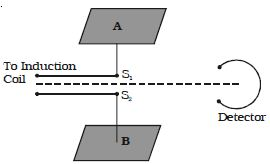# Describe Hertz Experiment of Electromagnetic Waves

Hertz experiment of electromagnetic waves

The existence of electromagnetic waves was confirmed experimentally by Hertz in 1888. This experiment is based on the fact that an oscillating electric charge radiates electromagnetic waves. The energy of these waves is due to the kinetic energy of the oscillating charge.

The experimental arrangement is as shown in Figure. It consists of two metal plates A and B placed at a distance of 60 cm from each other. The metal plates are connected to two polished metal spheres S1 and S2 by means of thick copper wires. Using an induction coil a high potential difference is applied across the small gap between the spheres.Fig; Hertz experiment

Due to high potential difference across S1 and S2, the air in the small gap between the spheres gets ionized and provides a path for the discharge of the plates. A spark is produced between S1 and S2 and electromagnetic waves of high frequency are radiated.

Hertz was able to produce electromagnetic waves of frequency about 5 × 107 Hz.

Here the plates A and B act as a capacitor having small capacitance value C and the connecting wires provide low inductance L. The high-frequency oscillation of charges between the plates is given by ν = 1/2π√LC